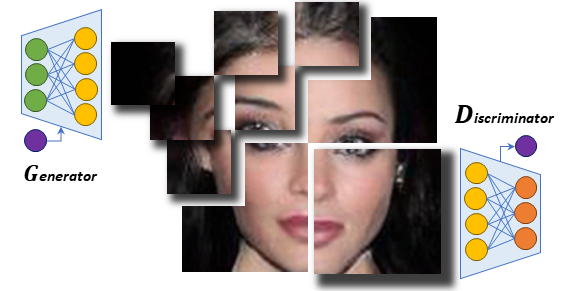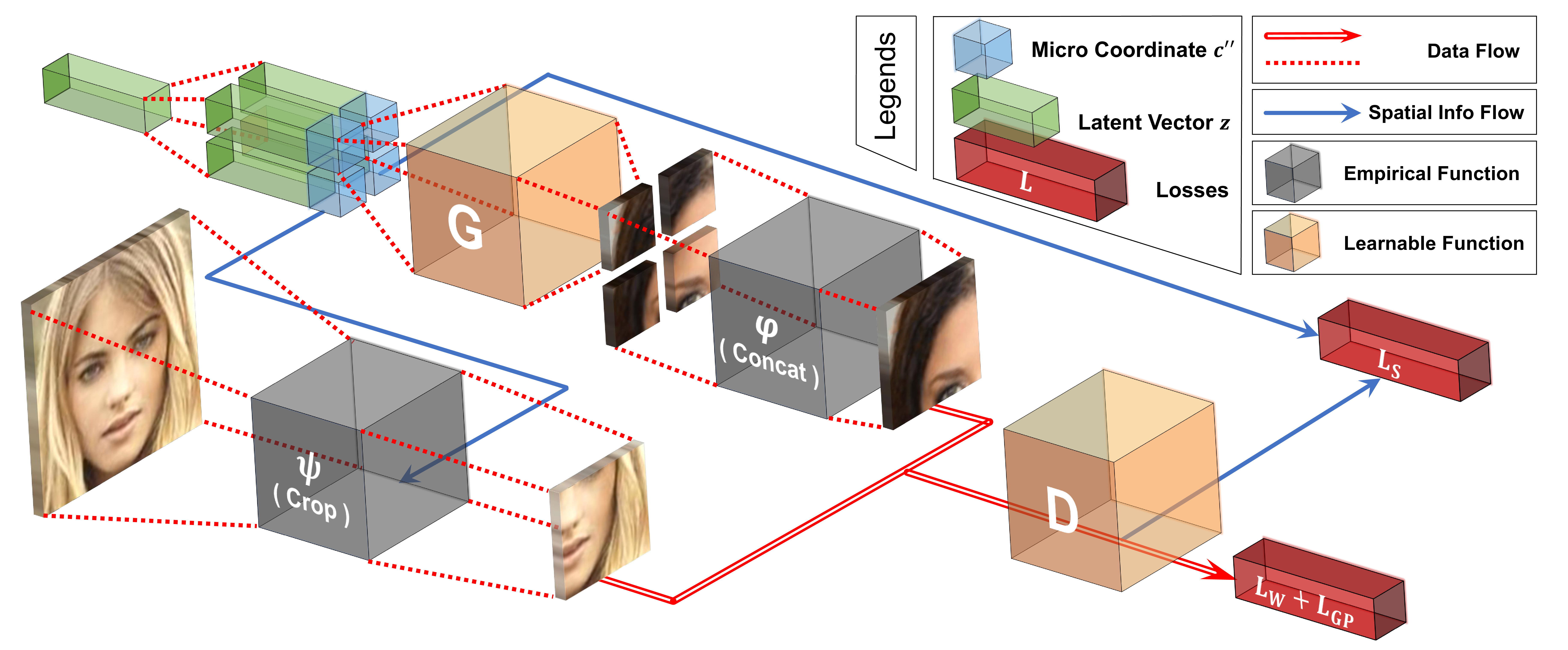# COCO-GAN: Generation by Parts via Conditional Coordinating - Chieh Hubert Lin - ICCV 2019

## Info

• Title: COCO-GAN: Generation by Parts via Conditional Coordinating
• Author: Chieh Hubert Lin, Chia-Che Chang, Yu-Sheng Chen, Da-Cheng Juan, Wei Wei, Hwann-Tzong Chen
• Date: Apr. 2019
• Arxiv: 1904.00284
• Published: ICCV 2019

## Highlights

• Discriminator with auxiliary task for content consistency(latent vector z and last featuremap from D) and spatial consistency(macro patch coordinates)

## Abstract

Humans can only interact with part of the surrounding environment due to biological restrictions. Therefore, we learn to reason the spatial relationships across a series of observations to piece together the surrounding environment. Inspired by such behavior and the fact that machines also have computational constraints, we propose COnditional COordinate GAN (COCO-GAN) of which the generator generates images by parts based on their spatial coordinates as the condition. On the other hand, the discriminator learns to justify realism across multiple assembled patches by global coherence, local appearance, and edge-crossing continuity. Despite the full images are never generated during training, we show that COCO-GAN can produce state-of-the-art-quality full images during inference. We further demonstrate a variety of novel applications enabled by teaching the network to be aware of coordinates. First, we perform extrapolation to the learned coordinate manifold and generate off-the-boundary patches. Combining with the originally generated full image, COCO-GAN can produce images that are larger than training samples, which we called “beyond-boundary generation”. We then showcase panorama generation within a cylindrical coordinate system that inherently preserves horizontally cyclic topology. On the computation side, COCO-GAN has a built-in divide-and-conquer paradigm that reduces memory requisition during training and inference, provides high-parallelism, and can generate parts of images on-demand.

## Motivation & DesignThe authors propose COnditional COordinate GAN (COCO-GAN) of which the generator generates images by parts based on their spatial coordinates as the condition. On the other hand, the discriminator learns to justify realism across multiple assembled patches by global coherence, local appearance, and edge-crossing continuity.For the COCO-GAN training, the latent vectors are duplicated multiple times, concatenated with micro coordinates, and feed to the generator to generate micro patches. Then we concatenate multiple micro patches to form a larger macro patch. The discriminator learns to discriminate between real and fake macro patches and an auxiliary task predicting the coordinate of the macro patch. Notice that none of the models requires full images during training.During the testing phase, the micro patches generated by the generator are directly combined into a full image as the final output. Still, none of the models requires full images. Furthermore, the generated images are high-quality without any post-processing in addition to a simple concatenation.

## Code

Project Page

### Model Graph

g_builder = GeneratorBuilder(config)
d_builder = DiscriminatorBuilder(config)
cp_builder = SpatialPredictorBuilder(config)
zp_builder = ContentPredictorBuilder(config)

def build_graph(self):

# Input nodes
# Note: the input node name was wrong in the checkpoint
self.micro_coord_fake = tf.placeholder(tf.float32, [None, self.spatial_dim], name='micro_coord_fake')
self.macro_coord_fake = tf.placeholder(tf.float32, [None, self.spatial_dim], name='macro_coord_fake')
self.micro_coord_real = tf.placeholder(tf.float32, [None, self.spatial_dim], name='micro_coord_real')
self.macro_coord_real = tf.placeholder(tf.float32, [None, self.spatial_dim], name='macro_coord_real')

# Reversing angle for cylindrical coordinate is complicated, directly pass values here
self.y_angle_ratio = tf.placeholder(tf.float32, [None, 1], name='y_angle_ratio')
self.z = tf.placeholder(tf.float32, [None, self.z_dim], name='z')

# Real part
self.real_macro = self.patch_handler.concat_micro_patches_gpu(
self.real_micro, ratio_over_micro=self.ratio_macro_to_micro)
(self.disc_real, disc_real_h) = self.d_builder(self.real_macro, self.macro_coord_real, is_training=True)
self.c_real_pred = self.cp_builder(disc_real_h, is_training=True)
self.z_real_pred = self.zp_builder(disc_real_h, is_training=True)

# Fake part
z_dup_macro = self._dup_z_for_macro(self.z)
self.gen_micro = self.g_builder(z_dup_macro, self.micro_coord_fake, is_training=True)
self.gen_macro = self.patch_handler.concat_micro_patches_gpu(
self.gen_micro, ratio_over_micro=self.ratio_macro_to_micro)
(self.disc_fake, disc_fake_h) = self.d_builder(self.gen_macro, self.macro_coord_fake, is_training=True)
self.c_fake_pred = self.cp_builder(disc_fake_h, is_training=True)
self.z_fake_pred = self.zp_builder(disc_fake_h, is_training=True)

# Patch-Guided Image Generation graph
if self._train_content_prediction_model():
(_, disc_real_h_rec) = self.d_builder(self.real_macro, None, is_training=False)
estim_z = self.zp_builder(disc_real_h_rec, is_training=False)
# I didn't especially handle this.
# if self.config["log_params"]["merge_micro_patches_in_cpu"]:
(_, self.rec_full) = self.generate_full_image_gpu(self.z)

print(" [Build] Composing Loss Functions ")
self._compose_losses()

print(" [Build] Creating Optimizers ")
self._create_optimizers()

def _compose_losses(self):

# Content consistency loss
self.code_loss = tf.reduce_mean(self.code_loss_w * tf.losses.absolute_difference(self.z, self.z_fake_pred))

# Spatial consistency loss (reduce later)
self.coord_mse_real = self.coord_loss_w * tf.losses.mean_squared_error(self.macro_coord_real, self.c_real_pred, reduction=NO_REDUCTION)
self.coord_mse_fake = self.coord_loss_w * tf.losses.mean_squared_error(self.macro_coord_fake, self.c_fake_pred, reduction=NO_REDUCTION)

self.coord_mse_real = tf.reduce_mean(self.coord_mse_real)
self.coord_mse_fake = tf.reduce_mean(self.coord_mse_fake)
self.coord_loss = self.coord_mse_real + self.coord_mse_fake

# WGAN loss

# Gradient penalty loss of WGAN-GP

# Total loss
self.d_loss = self.d_adv_loss + self.gp_loss + self.coord_loss + self.code_loss
self.g_loss = self.g_adv_loss + self.coord_loss + self.code_loss

# Wasserstein distance for visualization



snconv2d and snlinear are operators with Spectral Normalization.

### Generator

class GeneratorBuilder(Model):
def __init__(self, config):
self.config=config
self.ngf_base = self.config["model_params"]["ngf_base"]
self.num_extra_layers = self.config["model_params"]["g_extra_layers"]
self.micro_patch_size = self.config["data_params"]["micro_patch_size"]
self.c_dim = self.config["data_params"]["c_dim"]
self.update_collection = "G_update_collection"

def _cbn(self, x, y, is_training, scope=None):
with tf.variable_scope(scope, reuse=tf.AUTO_REUSE):
ch = x.shape.as_list()[-1]
gamma = slim.fully_connected(y, ch, activation_fn=None)
beta = slim.fully_connected(y, ch, activation_fn=None)

mean_rec = tf.get_variable("mean_recorder", [ch,],
initializer=tf.constant_initializer(np.zeros(ch)), trainable=False)
var_rec  = tf.get_variable("var_recorder", [ch,],
initializer=tf.constant_initializer(np.ones(ch)), trainable=False)
running_mean, running_var = tf.nn.moments(x, axes=[0, 1, 2])

if is_training:
new_mean_rec = 0.99 * mean_rec + 0.01 * running_mean
new_var_rec  = 0.99 * var_rec  + 0.01 * running_var
assign_mean_op = mean_rec.assign(new_mean_rec)
assign_var_op  = var_rec.assign(new_var_rec)
mean = running_mean
var  = running_var
else:
mean = mean_rec
var  = var_rec

# tiled_mean = tf.tile(tf.expand_dims(mean, 0), [tf.shape(x), 1])
# tiled_var  = tf.tile(tf.expand_dims(var , 0), [tf.shape(x), 1])
mean  = tf.reshape(mean, [1, 1, 1, ch])
var   = tf.reshape(var , [1, 1, 1, ch])
gamma = tf.reshape(gamma, [-1, 1, 1, ch])
beta  = tf.reshape(beta , [-1, 1, 1, ch])

out = (x-mean) / (var+_EPS) * gamma + beta
return out

def _g_residual_block(self, x, y, n_ch, idx, is_training, resize=True):
update_collection = self._get_update_collection(is_training)
with tf.variable_scope("g_resblock_"+str(idx), reuse=tf.AUTO_REUSE):
h = self._cbn(x, y, is_training, scope='g_resblock_cbn_1')
h = tf.nn.relu(h)
if resize:
h = upscale(h, 2)
h = snconv2d(h, n_ch, name='g_resblock_conv_1', update_collection=update_collection)
h = self._cbn(h, y, is_training, scope='g_resblock_cbn_2')
h = tf.nn.relu(h)
h = snconv2d(h, n_ch, name='g_resblock_conv_2', update_collection=update_collection)

if resize:
sc = upscale(x, 2)
else:
sc = x
sc = snconv2d(sc, n_ch, k_h=1, k_w=1, name='g_resblock_conv_sc', update_collection=update_collection)

return h + sc

def forward(self, z, coord, is_training):
valid_sizes = {4, 8, 16, 32, 64, 128, 256}
assert (self.micro_patch_size in valid_sizes and self.micro_patch_size in valid_sizes), \
"I haven't test your micro patch size: {}".format(self.micro_patch_size)

update_collection = self._get_update_collection(is_training)
print(" [Build] Generator ; is_training: {}".format(is_training))

with tf.variable_scope("G_generator", reuse=tf.AUTO_REUSE):
init_sp = 2
init_ngf_mult = 16
cond = tf.concat([z, coord], axis=1)
h = snlinear(cond, self.ngf_base*init_ngf_mult*init_sp*init_sp, 'g_z_fc', update_collection=update_collection)
h = tf.reshape(h, [-1, init_sp, init_sp, self.ngf_base*init_ngf_mult])

# Stacking residual blocks
num_resize_layers = int(math.log(min(self.micro_patch_size), 2) - 1)
num_total_layers  = num_resize_layers + self.num_extra_layers
basic_layers = [8, 4, 2]
if num_total_layers>=len(basic_layers):
num_replicate_layers = num_total_layers - len(basic_layers)
ngf_mult_list = basic_layers + [1, ] * num_replicate_layers
else:
ngf_mult_list = basic_layers[:num_total_layers]
print("\t ngf_mult_list = {}".format(ngf_mult_list))

for idx, ngf_mult in enumerate(ngf_mult_list):
n_ch = self.ngf_base * ngf_mult
# Standard layers first
if idx < num_resize_layers:
resize, is_extra = True, False
# Extra layers do not resize spatial size
else:
resize, is_extra = False, True
h = self._g_residual_block(h, cond, n_ch, idx=idx, is_training=is_training, resize=resize)
print("\t GResBlock: id={}, out_shape={}, resize={}, is_extra={}"
.format(idx, h.shape.as_list(), resize, is_extra))

h = batch_norm(name="g_last_bn")(h, is_training=is_training)
h = tf.nn.relu(h)
h = snconv2d(h, self.c_dim, name='g_last_conv_2', update_collection=update_collection)
return tf.nn.tanh(h)


### Discriminator

class DiscriminatorBuilder(Model):
def __init__(self, config):
self.config=config
self.ndf_base = self.config["model_params"]["ndf_base"]
self.num_extra_layers = self.config["model_params"]["d_extra_layers"]
self.macro_patch_size = self.config["data_params"]["macro_patch_size"]

self.update_collection = "D_update_collection"

def _d_residual_block(self, x, out_ch, idx, is_training, resize=True, is_head=False):
update_collection = self._get_update_collection(is_training)
with tf.variable_scope("d_resblock_"+str(idx), reuse=tf.AUTO_REUSE):
h = x
h = tf.nn.relu(h)
h = snconv2d(h, out_ch, name='d_resblock_conv_1', update_collection=update_collection)
h = tf.nn.relu(h)
h = snconv2d(h, out_ch, name='d_resblock_conv_2', update_collection=update_collection)
if resize:
h = slim.avg_pool2d(h, [2, 2])

# Short cut
s = x
if resize:
s = slim.avg_pool2d(s, [2, 2])
s = snconv2d(s, out_ch, k_h=1, k_w=1, name='d_resblock_conv_sc', update_collection=update_collection)
return h + s

def forward(self, x, y=None, is_training=True):
valid_sizes = {8, 16, 32, 64, 128, 256, 512}
assert (self.macro_patch_size in valid_sizes and self.macro_patch_size in valid_sizes), \
"I haven't test your macro patch size: {}".format(self.macro_patch_size)

update_collection = self._get_update_collection(is_training)
print(" [Build] Discriminator ; is_training: {}".format(is_training))

with tf.variable_scope("D_discriminator", reuse=tf.AUTO_REUSE):

num_resize_layers = int(math.log(min(self.macro_patch_size), 2) - 1)
num_total_layers  = num_resize_layers + self.num_extra_layers
basic_layers = [2, 4, 8, 8]
if num_total_layers>=len(basic_layers):
num_replicate_layers = num_total_layers - len(basic_layers)
ndf_mult_list = [1, ] * num_replicate_layers + basic_layers
else:
ndf_mult_list = basic_layers[-num_total_layers:]
print("\t ndf_mult_list = {}".format(ndf_mult_list))

# Stack extra layers without resize first
h = x
for idx, ndf_mult in enumerate(ndf_mult_list):
n_ch = self.ndf_base * ndf_mult
# Head is fixed and goes first
if idx==0:
is_head, resize, is_extra = True, True, False
# Extra layers before standard layers
elif idx<=self.num_extra_layers:
is_head, resize, is_extra = False, False, True
# Last standard layer has no resize
elif idx==len(ndf_mult_list)-1:
is_head, resize, is_extra = False, False, False
# Standard layers
else:
is_head, resize, is_extra = False, True, False

print("\t DResBlock: id={}, out_shape={}, resize={}, is_extra={}"
.format(idx, h.shape.as_list(), resize, is_extra))

h = tf.nn.relu(h)
h = tf.reduce_sum(h, axis=[1,2]) # Global pooling
last_feature_map = h
adv_out = snlinear(h, 1, 'main_steam_out', update_collection=update_collection)

# Projection Discriminator
if y is not None:
h_num_ch = self.ndf_base*ndf_mult_list[-1]
y_emb = snlinear(y, h_num_ch, 'y_emb', update_collection=update_collection)
proj_out = tf.reduce_sum(y_emb*h, axis=1, keepdims=True)
else:
proj_out = 0

return out, last_feature_map



### Spatial Prediction

class SpatialPredictorBuilder(Model):
def __init__(self, config):
self.config=config
self.aux_dim = config["model_params"]["aux_dim"]
self.spatial_dim = config["model_params"]["spatial_dim"]
self.update_collection = "GD_update_collection"

def forward(self, h, is_training):
print(" [Build] Spatial Predictor ; is_training: {}".format(is_training))
update_collection = self._get_update_collection(is_training)
h = snlinear(h, self.aux_dim, 'fc1', update_collection=update_collection)
h = batch_norm(name='bn1')(h, is_training=is_training)
h = lrelu(h)
h = snlinear(h, self.spatial_dim, 'fc2', update_collection=update_collection)
return tf.nn.tanh(h)


### Content Prediction

class ContentPredictorBuilder(Model):
def __init__(self, config):
self.config=config
self.z_dim = config["model_params"]["z_dim"]
self.aux_dim = config["model_params"]["aux_dim"]
self.update_collection = "Q_update_collection"

def forward(self, h, is_training):
print(" [Build] Spatial Predictor ; is_training: {}".format(is_training))
update_collection = self._get_update_collection(is_training)